# High School Math : Quadratic Equations and Inequalities

## Example Questions

1 2 3 4 5 7 Next →

### Example Question #3 : Using The Quadratic Formula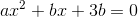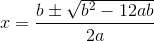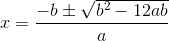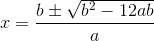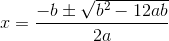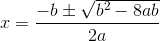Explanation:

Use the quadratic formula to solve: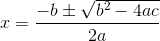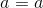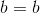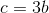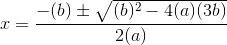### Example Question #4 : Using The Quadratic Formula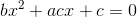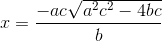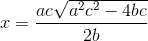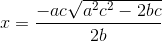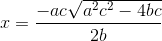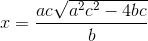Explanation:

Use the quadratic formula to solve: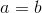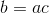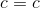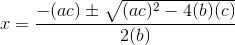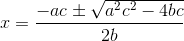### Example Question #5 : Using The Quadratic Formula

A baseball that is thrown in the air follows a trajectory of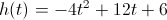, where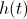is the height of the ball in feet andis the time elapsed in seconds. How long does the ball stay in the air before it hits the ground?

Between 3 and 3.5 seconds

Between 3.5 and 4 seconds

Between 2 and 2.5 seconds

Between 4 and 4.5 seconds

Between 2.5 and 3 seconds

Between 3 and 3.5 seconds

Explanation:

To solve this, we look at the equation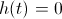.

Setting the equation equal to 0 we get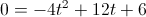.

Once in this form, we can use the Quadratic Formula to solve for.

The quadratic formula says that if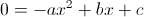, then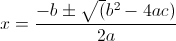.

Plugging in our values: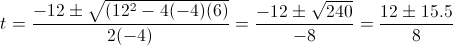Therefore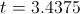or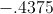and since we are looking only for positive values (because we can't have negative time), 3.4375 seconds is our answer.

### Example Question #41 : Solving Quadratic Equations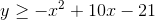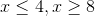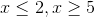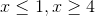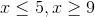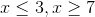Explanation:

Factor and solve.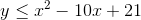Since the equation is less than or equal to, you know the inequality will be OR, not AND.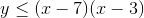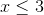or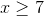### Example Question #42 : Solving Quadratic Equations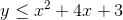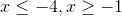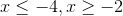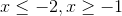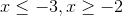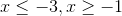Explanation:

Factor and solve. Since the sign is less than or equal to, we know the inequality will be OR, not AND.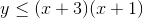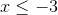or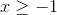### Example Question #43 : Solving Quadratic Equations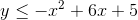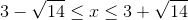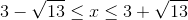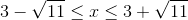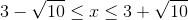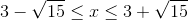Explanation:

Use the quadratic formula to solve.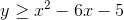Since the inequality is greater than or equal to, we know the inequality will be AND, not OR.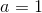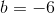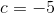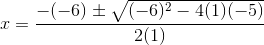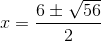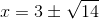1 2 3 4 5 7 Next →